# how to total a column in excel

Sum Range | Sum Entire Column | Sum Non-contiguous Cells | AutoSum | Sum Every Nth Row | Sum Largest Numbers | Sum Range with Errors

Use the SUM function in Excel to sum a range of cells, an entire column or non-contiguous cells. To create awesome SUM formulas, combine the SUM function with other Excel functions.

### Sum Range

Most of the time, you'll use the SUM function in Excel to sum a range of cells.### Sum Entire Column

You can also use the SUM function in Excel to sum an entire column.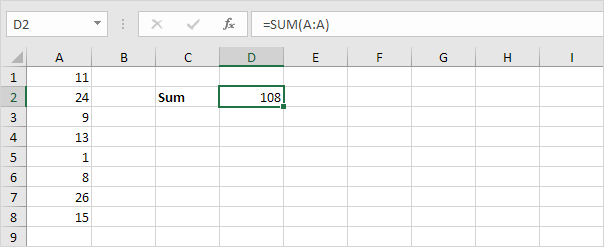Note: you can also use the SUM function in Excel to sum an entire row. For example, =SUM(5:5) sums all values in the 5th row.

### Sum Non-contiguous Cells

You can also use the SUM function in Excel to sum non-contiguous cells. Non-contiguous means not next to each other.Note: =A3+A5+A8 produces the exact same result!

### AutoSum

Use AutoSum or press ALT + = to quickly sum a column or row of numbers.

1. First, select the cell below the column of numbers (or next to the row of numbers) you want to sum.2. On the Home tab, in the Editing group, click AutoSum (or press ATL + =).3. Press Enter.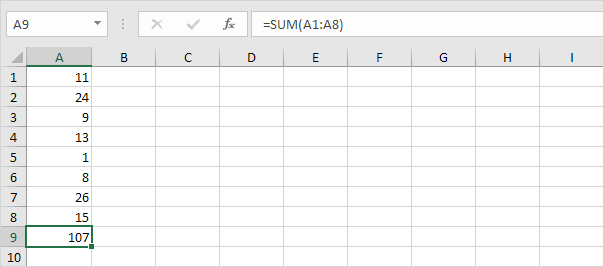### Sum Every Nth Row

The SUM formula below uses SUM, MOD and ROW to sum every nth row. Change the 3 to 4 to sum every 4th row, to 5 to sum every 5th row, etc.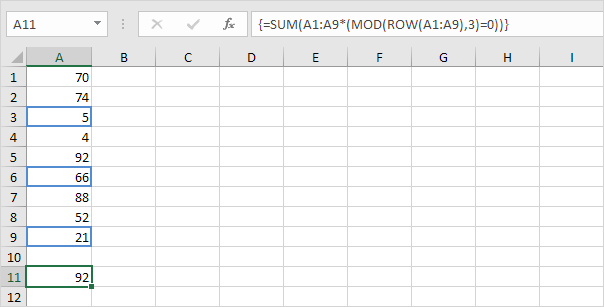Note: the formula bar indicates that this is an array formula by enclosing it in curly braces {}. Do not type these yourself. To enter an array formula, finish by pressing CTRL + SHIFT + ENTER.

### Sum Largest Numbers

The SUM formula below uses SUM and LARGE to sum the largest numbers in a range. Change {1,2,3,4} to {1,2,3,4,5} to sum the 5 largest numbers.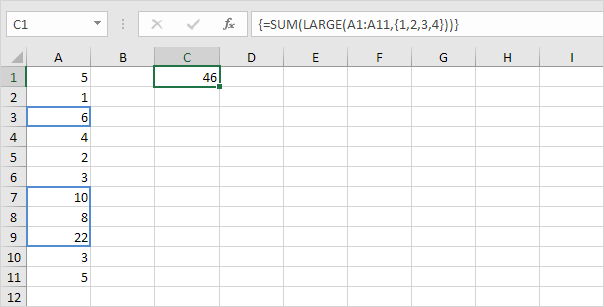Note: =LARGE(A1:A11,2) simply returns the second largest number.

### Sum Range with Errors

The SUM formula below uses SUM and IFERROR to sum a range with errors. You can also use the AGGREGATE function in Excel to sum a range with errors.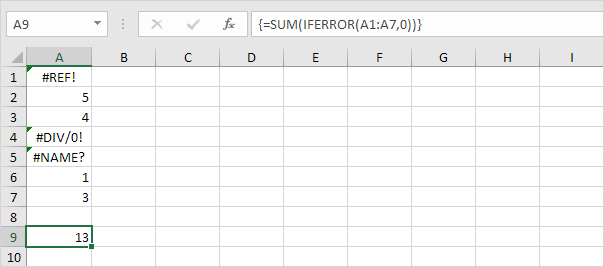Note: the SUM function in Excel automatically ignores text values.